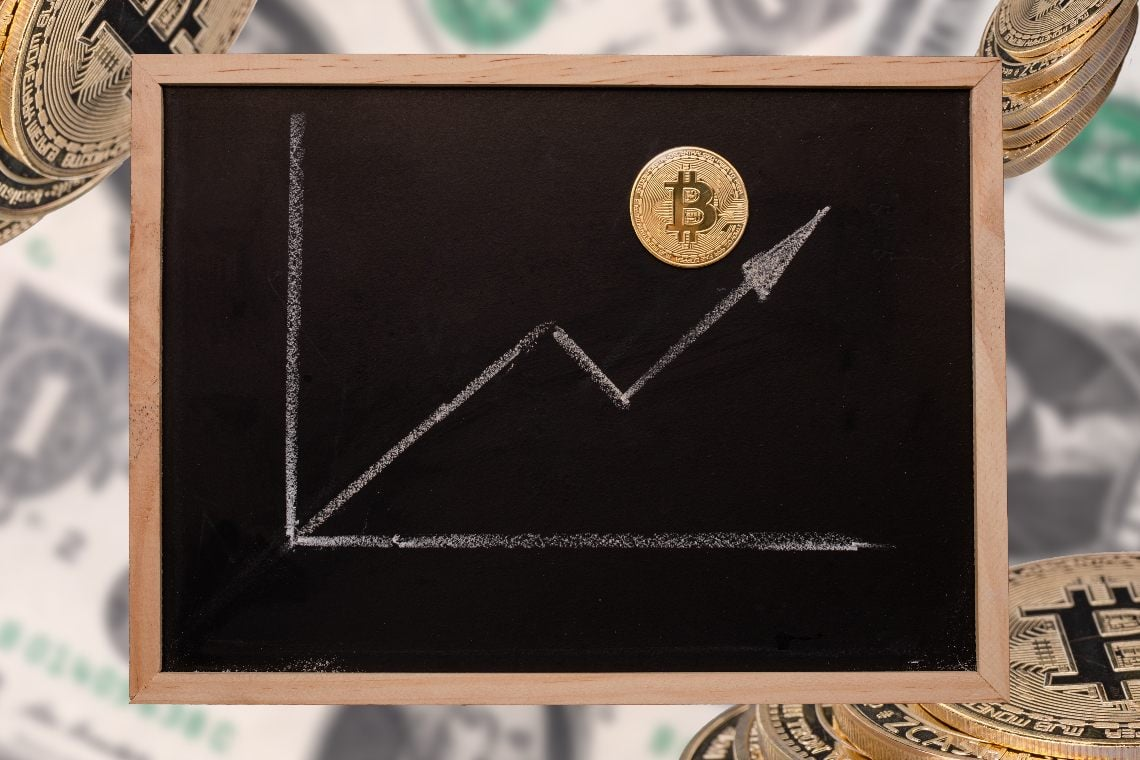Bitcoin

# Bitcoin: Fibonacci extension indicates possible strong growth

A recent analysis of bitcoin’s historical price based on the Fibonacci Channel extension indicates possible further growth.

The analysis was published on TradingView by TradingShot, and shows the logarithmic chart of the bitcoin price from 2010 to the present with Fibonacci Channel extensions.

By dividing the curve into four cycles, which are equal to the current halving cycles, for each cycle the Fibonacci Channel extensions are calculated.

In the first cycle, which ended in mid-2011, only two extensions are given, in the second cycle, which ended in December 2013, three extensions are given, in the third cycle, which ended in January 2018, four extensions are given, and for the fourth cycle it is assumed that there may be five extensions.

Right now the price of bitcoin, during the fourth cycle that began in December 2018, would be around the third extension, with the fourth pointing to \$300,000 in November 2022, and the fifth to \$700,000 in December 2022.

## The positive forecast of the Fibonacci Channel

Looking only at 2021, the fourth Fib Channel extension would point to a price of around \$200,000 and the fifth to a price of around \$450,000, which is similar to Bloomberg Intelligence’s prediction if a trend similar to 2013 or 2017 were to repeat in 2021.

TradingShot writes that, using the parabolic growth curve, and Fibonacci Channel extensions, the maximum price of BTC reached during the first cycle was just below the 2.0 extension, while that of the second cycle was exactly on the 3.0 extension, and that of the third cycle was just below the 4.0 extension.

At this point he writes:

“As you realize the pattern is arithmetic and every Cyclical Top is one Fib higher. Naturally the mind assumes that on Bitcoin’s current (4th) Cycle, the Top could be on the 5.0 Fibonacci extension”.